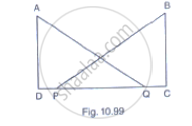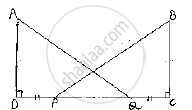Share
Notifications

View all notifications
Books Shortlist
Your shortlist is empty

# In Fig. 10.99, Ad ⊥ Cd and Cb ⊥. Cd. If Aq = Bp and Dp = Cq, Prove that ∠Daq = ∠Cbp. - CBSE Class 9 - Mathematics

Login
Create free account

Forgot password?
ConceptCriteria for Congruence of Triangles

#### Question

In Fig. 10.99, AD ⊥ CD and CB ⊥. CD. If AQ = BP and DP = CQ, prove that ∠DAQ = ∠CBP.#### Solution

Given that, in the figure AD ⊥ CD and CB ⊥ CD and AQ = BP,DP =CQ

We have to prove that ∠DAQ=∠CBP

Given that DP= QC

Add PQ on both sides

Given that DP=QC

Add PQ on both sides

⇒ DP+PQ=PQ+QC

⇒ DQ=PC                 ................(1)

Now, consider triangle DAQ and CBP,
We have

∠ADQ=∠BCP=90°            [given]

AQ=BP                           [given]

And DQ=PC                   [given]

So, by RHS congruence criterion, we have ΔDAQ≅ΔCBP

Now,

∠DAQ=∠CBP         [ ∵Corresponding parts of congruent triangles are equal]

∴ Hence provedIs there an error in this question or solution?

#### APPEARS IN

Solution In Fig. 10.99, Ad ⊥ Cd and Cb ⊥. Cd. If Aq = Bp and Dp = Cq, Prove that ∠Daq = ∠Cbp. Concept: Criteria for Congruence of Triangles.
S